# SimpleGenericOrifice

Simple generic orifice defined by pressure loss coefficient and diameter (only for flow from port_a to port_b)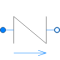# Information

This information is part of the Modelica Standard Library maintained by the Modelica Association.

This pressure drop component defines a simple, generic orifice, where the loss factor ζ is provided for one flow direction (e.g., from loss table of a book):

```   Δp = 0.5*ζ*ρ*v*|v|
= 8*ζ/(π^2*D^4*ρ) * m_flow*|m_flow|
```

where

• Δp is the pressure drop: Δp = port_a.p - port_b.p
• D is the diameter of the orifice at the position where ζ is defined (either at port_a or port_b). If the orifice has not a circular cross section, D = 4*A/P, where A is the cross section area and P is the wetted perimeter.
• ζ is the loss factor with respect to D that depends on the geometry of the orifice. In the turbulent flow regime, it is assumed that ζ is constant.
For small mass flow rates, the flow is laminar and is approximated by a polynomial that has a finite derivative for m_flow=0.
• v is the mean velocity.
• ρ is the upstream density.

Since the pressure loss factor zeta is provided only for a mass flow from port_a to port_b, the pressure loss is not correct when the flow is reversing. If reversing flow only occurs in a short time interval, this is most likely uncritical. If significant reversing flow can appear, this component should not be used.

# Parameters (14)

dp_start Value: dp_nominal Type: AbsolutePressure (Pa) Description: Guess value of dp = port_a.p - port_b.p Value: if system.use_eps_Re then system.eps_m_flow * m_flow_nominal else system.m_flow_small Type: MassFlowRate (kg/s) Description: Small mass flow rate for regularization of zero flow Value: true Type: Boolean Description: = true, if temperatures at port_a and port_b are computed Value: true Type: Boolean Description: = true, if volume flow rate at inflowing port is computed Value: system.allowFlowReversal Type: Boolean Description: = true to allow flow reversal, false restricts to design direction (m_flow >= 0) Value: Types.Dynamics.SteadyState Type: Dynamics Description: Formulation of momentum balance Value: system.m_flow_start Type: MassFlowRate (kg/s) Description: Start value of mass flow rates Value: Type: Diameter (m) Description: Diameter of orifice Value: Type: Real Description: Loss factor for flow of port_a -> port_b Value: true Type: Boolean Description: = false to obtain zeta from dp_nominal and m_flow_nominal Value: if system.use_eps_Re then system.m_flow_nominal else 1e2 * system.m_flow_small Type: MassFlowRate (kg/s) Description: Mass flow rate for dp_nominal Value: if not system.use_eps_Re then 1e3 else BaseClasses.lossConstant_D_zeta(diameter, zeta) / Medium.density_pTX(Medium.p_default, Medium.T_default, Medium.X_default) * m_flow_nominal ^ 2 Type: Pressure (Pa) Description: Nominal pressure drop Value: system.use_eps_Re Type: Boolean Description: = true, if turbulent region is defined by Re, otherwise by m_flow_small Value: true Type: Boolean Description: = true, use m_flow = f(dp) else dp = f(m_flow)

# Inputs (1)

pathLength Default Value: 0 Type: Length (m) Description: Length flow path

# Connectors (2)

port_a port_b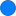Type: FluidPort_a Description: Fluid connector a (positive design flow direction is from port_a to port_b)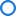Type: FluidPort_b Description: Fluid connector b (positive design flow direction is from port_a to port_b)

# Components (3)

state_a state_b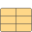Type: ThermodynamicState Description: state for medium inflowing through port_aType: ThermodynamicState Description: state for medium inflowing through port_b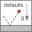Type: System Description: System properties

# Used in Examples (1)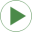InverseParameterization Modelica.Fluid.Examples Demonstrates the parameterization of a pump and a pipe for given nominal values